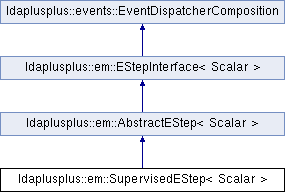LDA++
ldaplusplus::em::SupervisedEStep< Scalar > Class Template Reference

#include <SupervisedEStep.hpp>

Inheritance diagram for ldaplusplus::em::SupervisedEStep< Scalar >:## Public Member Functions

SupervisedEStep (size_t e_step_iterations=10, Scalar e_step_tolerance=1e-2, size_t fixed_point_iterations=20, Scalar compute_likelihood=1.0, int random_state=0)

std::shared_ptr< parameters::Parametersdoc_e_step (const std::shared_ptr< corpus::Document > doc, const std::shared_ptr< parameters::Parameters > parameters) overridePublic Member Functions inherited from ldaplusplus::em::AbstractEStep< Scalar >
AbstractEStep (int random_state)

virtual void e_step () overridePublic Member Functions inherited from ldaplusplus::events::EventDispatcherComposition
std::shared_ptr< EventDispatcherInterfaceget_event_dispatcher ()

void set_event_dispatcher (std::shared_ptr< EventDispatcherInterface > dispatcher)Protected Member Functions inherited from ldaplusplus::em::AbstractEStep< Scalar >
bool converged (const Eigen::Matrix< Scalar, Eigen::Dynamic, 1 > &gamma_old, const Eigen::Matrix< Scalar, Eigen::Dynamic, 1 > &gamma, Scalar tolerance)

PRNGget_prng ()

## Detailed Description

### template<typename Scalar> class ldaplusplus::em::SupervisedEStep< Scalar >

SupervisedEStep implements the categorical supervised LDA expectation step.

As in UnsupervisedEStep the parameters of a distribution ( $$\gamma$$ and $$\phi$$) are computed such that the likelihood of generating each document is maximized. For all supervised LDA variants except for generating the document the likelihood of generating the label is also maximized.

The solution implemented in SupervisedEStep is the one presented in paper .

 Mcauliffe, Jon D., and David M. Blei. "Supervised topic models." Advances in neural information processing systems. 2008

 Chong, Wang, David Blei, and Fei-Fei Li. "Simultaneous image classification and annotation." Computer Vision and Pattern Recognition, 2009 CVPR 2009 IEEE Conference on. IEEE, 2009

## Constructor & Destructor Documentation

template<typename Scalar >
 ldaplusplus::em::SupervisedEStep< Scalar >::SupervisedEStep ( size_t e_step_iterations = 10, Scalar e_step_tolerance = 1e-2, size_t fixed_point_iterations = 20, Scalar compute_likelihood = 1.0, int random_state = 0 )
Parameters
 e_step_iterations The max number of times to alternate between maximizing for $$\gamma$$ and for $$\phi$$. e_step_tolerance The minimum relative change in the variational parameter $$\gamma$$. fixed_point_iterations The number of fixed point iterations used in the maximization for $$\phi$$. compute_likelihood The percentage of documents to compute likelihood for (1.0 means compute for every document) random_state An initial seed value for any random numbers needed

## Member Function Documentation

template<typename Scalar >
 std::shared_ptr< parameters::Parameters > ldaplusplus::em::SupervisedEStep< Scalar >::doc_e_step ( const std::shared_ptr< corpus::Document > doc, const std::shared_ptr< parameters::Parameters > parameters )
overridevirtual

Maximize the ELBO w.r.t. to $$\phi$$ and $$\gamma$$.

The following steps are the mathematics that are implemented where $$\beta$$ are the topics, $$\eta$$ are the logistic regression parameters, $$i$$ is the topic subscript, $$n$$ is the word subscript, $$\hat{y}$$ is the class subscript, $$y$$ is the document's class, $$w_n$$ is n-th word vocabulary index, $$\alpha$$ is the Dirichlet prior and finally $$\Psi(\cdot)$$ is the first derivative of the $$\log \Gamma$$ function.

1. Repeat until convergence
1. Repeat for fixed_point_iterations
2. Compute $$h$$ such that $$h^T \phi_n = \sum_{\hat y}^C \prod_n^N \left( \sum_i^K \phi_{ni} \exp(\frac{1}{N}\eta_{\hat{y}i}) \right)$$ and $$h$$ doesn't contain $$\phi_n$$
3. $$\phi_{ni} \propto \beta_{iw_n} \exp\left( \Psi(\gamma_i) + \frac{1}{N} \eta_{yi} + \frac{h_i}{h^T\phi_n^{\text{old}}} \right)$$
2. $$\gamma_i = \alpha_i + \sum_n^N \phi_{ni}$$
Parameters
 doc A single document parameters An instance of class Parameters, which contains all necessary model parameters for e-step's implementation
Returns
The variational parameters for the current model, after e-step is completed

Implements ldaplusplus::em::EStepInterface< Scalar >.

The documentation for this class was generated from the following files: